您可以捐助，支持我们的公益事业。 1元 10元 50元 认证码：必填求知 文章 文库 Lib 视频 iPerson 课程 认证 咨询 工具 讲座 Modeler Code要资料订阅捐助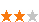382 次浏览     评价： 好 中 差
2019-11-25

 编辑推荐: 文章介绍了如何建立起一个任意层数的深度神经网络。这个神经网络可以应用于二元分类的监督学习问题。 本文来自于百度，由火龙果软件Alice编辑、推荐。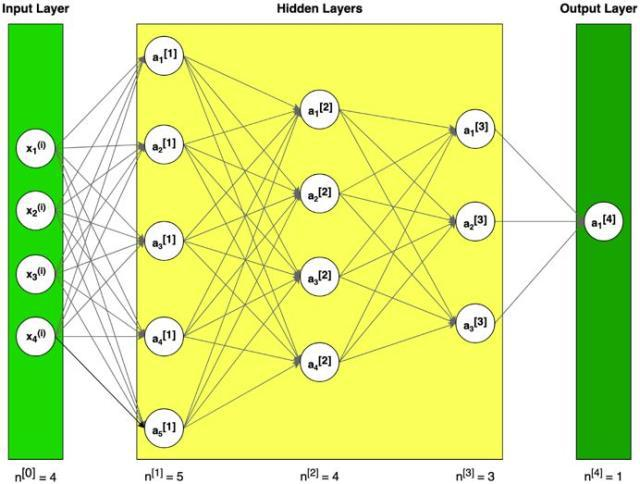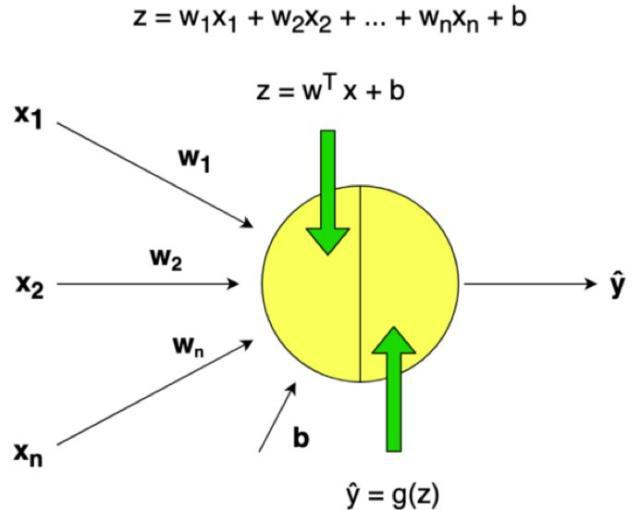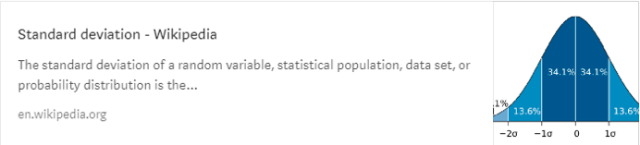1. 定义模型构造（例如，数据的输入特征）

2. 初始化参数并定义超参数

3. 迭代循环

4. 使用训练参数来预测标签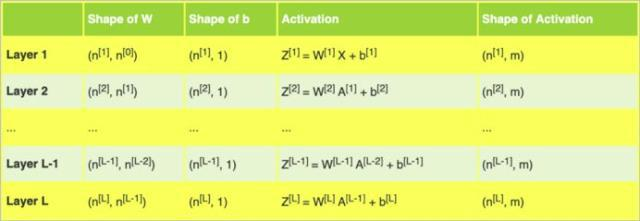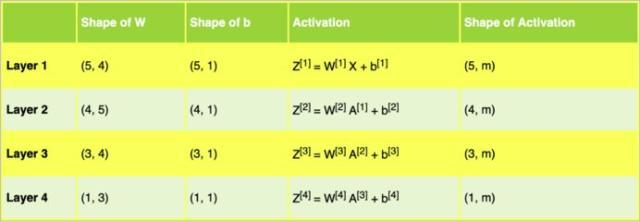import numpy as np import matplotlib.pyplot as plt nn_architecture = [{"layer_size": 4,"activation": "none"}, # input layer{"layer_size": 5,"activation": "relu"},{"layer_size": 4,"activation": "relu"},{"layer_size": 3,"activation": "relu"},{"layer_size": 1,"activation": "sigmoid"}]def initialize_parameters(nn_architecture, seed = 3):np.random.seed(seed)# python dictionary containingour parameters "W1", "b1", ..., "WL","bL"parameters = {}number_of_layers = len(nn_architecture)for l in range(1,number_of_layers):parameters['W' + str(l)] =np.random.randn(nn_architecture[l]["layer_size"],nn_architecture[l-1]["layer_size"]) * 0.01parameters['b' + str(l)] =np.zeros((nn_architecture[l]["layer_size"], 1))return parameters

・ 不同的初始化工具，例如Zero,Random, He or Xavier，都会导致不同的结果。

・ 随机初始化能够确保不同的隐藏单元可以学习不同的东西（初始化所有权重为零会导致，所有层次的所有感知机都将学习相同的东西）。

・ 不要初始化为太大的值。

Sigmoid输出一个介于0和1之间的值，这使得它成为二进制分类的一个很好的选择。如果输出小于0.5，可以将其分类为0；如果输出大于0.5，可以将其分类为1。

 def sigmoid(Z): S = 1 / (1 + np.exp(-Z))return Sdef relu(Z):R = np.maximum(0, Z)return Rdef sigmoid_backward(dA, Z):S = sigmoid(Z)dS = S * (1 - S)return dA * dSdef relu_backward(dA, Z):dZ = np.array(dA, copy = True)dZ[Z <= 0] = 0return dZ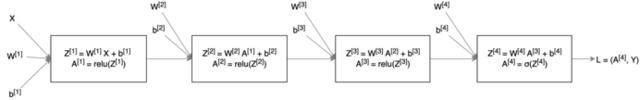def L_model_forward(X, parameters, nn_architecture): forward_cache = {}A = Xnumber_of_layers =len(nn_architecture)for l in range(1,number_of_layers):A_prev = AW = parameters['W' + str(l)]b = parameters['b' + str(l)]activation =nn_architecture[l]["activation"]Z, A =linear_activation_forward(A_prev, W, b, activation)forward_cache['Z' + str(l)] =Zforward_cache['A' + str(l)] =AAL = Areturn AL, forward_cachedef linear_activation_forward(A_prev, W, b, activation):if activation =="sigmoid":Z = linear_forward(A_prev, W,b)A = sigmoid(Z)elif activation =="relu":Z = linear_forward(A_prev, W,b)A = relu(Z)return Z, Adef linear_forward(A, W, b):Z = np.dot(W, A) + breturn Z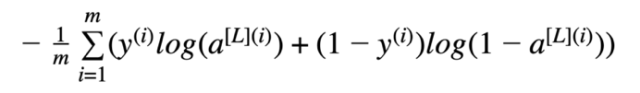def compute_cost(AL, Y): m = Y.shape# Compute loss from AL and ylogprobs =np.multiply(np.log(AL),Y) + np.multiply(1 - Y, np.log(1 - AL))# cross-entropy costcost = - np.sum(logprobs) / mcost = np.squeeze(cost)return cost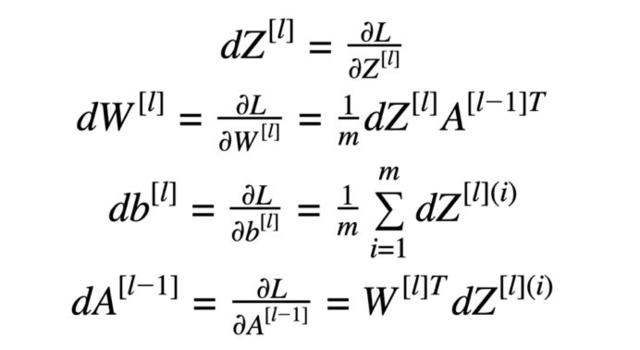“链规则”在计算损失时十分重要（以方程式5为例）。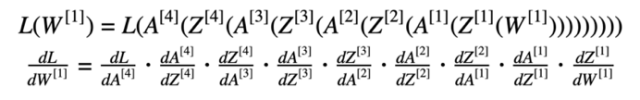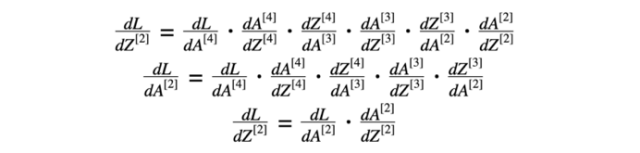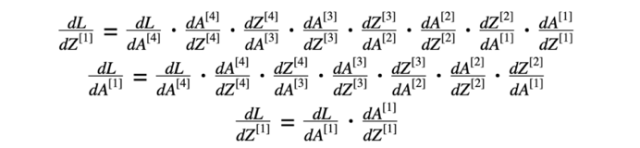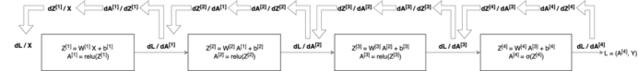def L_model_backward(AL, Y, parameters, forward_cache, nn_architecture): grads = {}number_of_layers =len(nn_architecture)m = AL.shapeY = Y.reshape(AL.shape) # afterthis line, Y is the same shape as AL# Initializing thebackpropagationdAL = - (np.divide(Y, AL) -np.divide(1 - Y, 1 - AL))dA_prev = dALfor l in reversed(range(1,number_of_layers)):dA_curr = dA_prevactivation =nn_architecture[l]["activation"]W_curr = parameters['W' +str(l)]Z_curr = forward_cache['Z' +str(l)]A_prev = forward_cache['A' +str(l-1)]dA_prev, dW_curr, db_curr =linear_activation_backward(dA_curr, Z_curr, A_prev, W_curr, activation)grads["dW" +str(l)] = dW_currgrads["db" +str(l)] = db_currreturn gradsdef linear_activation_backward(dA, Z, A_prev, W, activation):if activation =="relu":dZ = relu_backward(dA, Z)dA_prev, dW, db =linear_backward(dZ, A_prev, W)elif activation =="sigmoid":dZ = sigmoid_backward(dA, Z)dA_prev, dW, db =linear_backward(dZ, A_prev, W)return dA_prev, dW, dbdef linear_backward(dZ, A_prev, W):m = A_prev.shapedW = np.dot(dZ, A_prev.T) / mdb = np.sum(dZ, axis=1,keepdims=True) / mdA_prev = np.dot(W.T, dZ)return dA_prev, dW, db

 def update_parameters(parameters, grads, learning_rate): L = len(parameters)for l in range(1, L):parameters["W" +str(l)] = parameters["W" + str(l)] - learning_rate *grads["dW" + str(l)]parameters["b" +str(l)] = parameters["b" + str(l)] - learning_rate *grads["db" + str(l)]return parameters

 def L_layer_model(X, Y, nn_architecture, learning_rate = 0.0075,num_iterations = 3000, print_cost=False): np.random.seed(1)# keep track of costcosts = []# Parameters initialization.parameters =initialize_parameters(nn_architecture)# Loop (gradient descent)for i in range(0,num_iterations):# Forward propagation:[LINEAR -> RELU]*(L-1) -> LINEAR -> SIGMOID.AL, forward_cache =L_model_forward(X, parameters, nn_architecture)# Compute cost.cost = compute_cost(AL, Y)# Backward propagation.grads = L_model_backward(AL,Y, parameters, forward_cache, nn_architecture)# Update parameters.parameters =update_parameters(parameters, grads, learning_rate)# Print the cost every 100training exampleif print_cost and i % 100 ==0:print("Cost afteriteration %i: %f" %(i, cost))costs.append(cost)# plot the costplt.plot(np.squeeze(costs))plt.ylabel('cost')plt.xlabel('iterations (pertens)')plt.title("Learning rate=" + str(learning_rate))plt.show()return parameters

・ 小批量梯度下降

・ 动力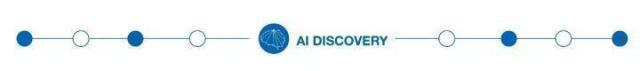382 次浏览     评价： 好 中 差订阅捐助